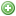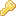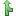FAQSearchRegisterLogin

## Wave patterns

Related to the development libraries, released by Curt BinderrimaiPosts: 12716Joined: Fri Mar 18, 2011 6:47 pmPosted: Thu Mar 21, 2013 12:38 pm I'd like to start a thread to collect all the efforts of creating wave patterns for controllable pumps.These functions can be used for either Tunzes or Jebao pumps.This list will keep being updated as we code more and more functions.Short PulsePulseMinSpeed - % for minimal speedPulseMaxSpeed - % for maximum speedPulseDuration - Duration (milliseconds) in which each pulse will be held. The pump will stay at minimal speed for PulseDuration and will stay at maximum speed for PulseDuration.PulseSync - true if you want to sync pumps to same cycle. one false and one true if you want to anti-sync pumps.Code: Select all`byte ShortPulseMode(byte PulseMinSpeed, byte PulseMaxSpeed, int PulseDuration, boolean PulseSync){  byte tspeed=0;  PulseMinSpeed=constrain(PulseMinSpeed,30,100);  PulseMaxSpeed=constrain(PulseMaxSpeed,30,100);  tspeed=(millis()%(PulseDuration*2) 5000)  {    if (random(100)<50) newspeed--; else newspeed++;    newspeed=constrain(newspeed,WaveSpeed-WaveOffset,WaveSpeed+WaveOffset);    newspeed=constrain(newspeed,0,100);    lastwavemillis=millis();  }    if (PulseSync)    return newspeed;  else    return WaveSpeed-(newspeed-WaveSpeed);}`Nutrient TransportPulseMinSpeed - % for minimal speedPulseMaxSpeed - % for maximum speedPulseDuration - Duration (milliseconds) in which each pulse will be held. The pump will stay at minimal speed for PulseDuration and will stay at maximum speed for PulseDuration.PulseSync - true if you want to sync pumps to same cycle. one false and one true if you want to anti-sync pumps.Code: Select all`byte NutrientTransportMode(byte PulseMinSpeed, byte PulseMaxSpeed, int PulseDuration, boolean PulseSync){  static unsigned long lastwavemillis=millis();  static byte WavePhase=0;  static time_t WaveStart=0;  static byte speed=PulseMinSpeed;  static byte anti_speed=PulseMinSpeed;  if (WavePhase==0)  {    WavePhase++;    WaveStart=now();  }  else if (WavePhase==1)  {    if (now()-WaveStart>2700)    {      WavePhase++;    }    if ((millis()-lastwavemillis) > PulseDuration)    {      if (speed==PulseMinSpeed)      {          speed=PulseMaxSpeed;        anti_speed=PulseMinSpeed;      }      else      {        speed=PulseMinSpeed;        anti_speed=PulseMaxSpeed;      }      lastwavemillis=millis();    }  }  else if (WavePhase==2)  {    if (now()-WaveStart>4500) WavePhase++;    if (now()-WaveStart<=2760)      speed=PulseMinSpeed;     else      speed=PulseMaxSpeed;    if (now()-WaveStart<=3300)      anti_speed=PulseMinSpeed;    else      anti_speed=PulseMaxSpeed*sin(radians(map(now()-WaveStart,3300,4500,0,180)));  }  else if (WavePhase==3)  {    if (now()-WaveStart>7200) WavePhase++;    if ((millis()-lastwavemillis) > PulseDuration)    {      if (speed==PulseMinSpeed)      {          speed=PulseMaxSpeed;        anti_speed=PulseMinSpeed;      }      else      {        speed=PulseMinSpeed;        anti_speed=PulseMaxSpeed;      }      lastwavemillis=millis();    }  }  else if (WavePhase==4)  {    if (now()-WaveStart>9000) WavePhase=0;    if (now()-WaveStart<=7260)       speed=PulseMinSpeed;     else      speed=PulseMaxSpeed;    if (now()-WaveStart<=8400)      anti_speed=PulseMaxSpeed*sin(radians(map(now()-WaveStart,7200,8400,0,180)));    else      anti_speed=0;  }  if (PulseSync)    return speed;  else    return anti_speed;}`Tidal Swell ModeWaveMaxSpeed - % for maximum speedPulseSync - true if you want to sync pumps to same cycle. one false and one true if you want to anti-sync pumps.Code: Select all`byte TidalSwellMode(byte WaveMaxSpeed, boolean PulseSync){  static unsigned long lastwavemillis=millis();  static byte WavePhase=0;  static time_t WaveStart=0;  static byte speed=0;  static byte anti_speed=0;  if (WavePhase==0)  {    WavePhase++;    WaveStart=now();  }  else if (WavePhase==1)  {    if (now()-WaveStart>900) WavePhase++;    speed=(WaveMaxSpeed*sin(radians(map(now()-WaveStart,0,900,0,90))))/10;    speed+=WaveMaxSpeed/2;    anti_speed=(WaveMaxSpeed*2*sin(radians(map(now()-WaveStart,0,900,0,90))))/5;    anti_speed+=WaveMaxSpeed/2;  }  else if (WavePhase==2)  {    if (now()-WaveStart>1800) WavePhase++;    speed=(WaveMaxSpeed*sin(radians(map(now()-WaveStart,900,1800,90,180))))/20;    speed+=WaveMaxSpeed/2;    speed+=WaveMaxSpeed/20;    anti_speed=(WaveMaxSpeed*3*sin(radians(map(now()-WaveStart,900,1800,90,180))))/20;    anti_speed+=WaveMaxSpeed/2;    anti_speed+=WaveMaxSpeed/4;  }  else if (WavePhase==3)  {    if (now()-WaveStart>2700) WavePhase++;    speed=(WaveMaxSpeed*3*sin(radians(map(now()-WaveStart,1800,2700,0,90))))/20;    speed+=WaveMaxSpeed/2;    speed+=WaveMaxSpeed/20;    anti_speed=(WaveMaxSpeed*sin(radians(map(now()-WaveStart,1800,2700,0,90))))/20;    anti_speed+=WaveMaxSpeed/2;    anti_speed+=WaveMaxSpeed/4;  }  else if (WavePhase==4)  {    if (now()-WaveStart>3600) WavePhase++;    speed=(WaveMaxSpeed*sin(radians(map(now()-WaveStart,2700,3600,90,180))))/20;    speed+=WaveMaxSpeed/2;    speed+=(WaveMaxSpeed*3)/20;    anti_speed=(WaveMaxSpeed*3*sin(radians(map(now()-WaveStart,2700,3600,90,180))))/20;    anti_speed+=WaveMaxSpeed/2;    anti_speed+=(WaveMaxSpeed*3)/20;  }  else if (WavePhase==5)  {    if (now()-WaveStart>4500) WavePhase++;    speed=(WaveMaxSpeed*3*sin(radians(map(now()-WaveStart,3600,4500,0,90))))/20;    speed+=WaveMaxSpeed/2;    speed+=(WaveMaxSpeed*3)/20;    anti_speed=(WaveMaxSpeed*sin(radians(map(now()-WaveStart,3600,4500,0,90))))/20;    anti_speed+=WaveMaxSpeed/2;    anti_speed+=(WaveMaxSpeed*3)/20;  }  else if (WavePhase==6)  {    if (now()-WaveStart>5400) WavePhase++;    speed=(WaveMaxSpeed*sin(radians(map(now()-WaveStart,4500,5400,90,180))))/20;    speed+=WaveMaxSpeed/2;    speed+=(WaveMaxSpeed*5)/20;    anti_speed=(WaveMaxSpeed*3*sin(radians(map(now()-WaveStart,4500,5400,90,180))))/20;    anti_speed+=WaveMaxSpeed/2;    anti_speed+=WaveMaxSpeed/20;  }  else if (WavePhase==7)  {    if (now()-WaveStart>6300) WavePhase++;    speed=(WaveMaxSpeed*3*sin(radians(map(now()-WaveStart,5400,6300,0,90))))/20;    speed+=WaveMaxSpeed/2;    speed+=(WaveMaxSpeed*5)/20;    anti_speed=(WaveMaxSpeed*sin(radians(map(now()-WaveStart,5400,6300,0,90))))/20;    anti_speed+=WaveMaxSpeed/2;    anti_speed+=WaveMaxSpeed/20;  }  else if (WavePhase==8)  {    if (now()-WaveStart>7200) WavePhase++;    speed=(WaveMaxSpeed*sin(radians(map(now()-WaveStart,6300,7200,90,180))))/20;    speed+=WaveMaxSpeed/2;    speed+=(WaveMaxSpeed*7)/20;    anti_speed=(WaveMaxSpeed*sin(radians(map(now()-WaveStart,6300,7200,90,180))))/10;    anti_speed+=WaveMaxSpeed/2;  }  else if (WavePhase==9)  {    if (now()-WaveStart>8100) WavePhase++;    speed=(WaveMaxSpeed*3*sin(radians(map(now()-WaveStart,7200,8100,0,90))))/20;    speed+=WaveMaxSpeed/2;    speed+=(WaveMaxSpeed*7)/20;    anti_speed=(WaveMaxSpeed*sin(radians(map(now()-WaveStart,7200,8100,0,90))))/2;    anti_speed+=WaveMaxSpeed/2;  }  else if (WavePhase==10)  {    if (now()-WaveStart>9000) WavePhase=0;    speed=(WaveMaxSpeed*sin(radians(map(now()-WaveStart,8100,9000,90,180))))/2;    speed+=WaveMaxSpeed/2;    anti_speed=speed;  }  if (PulseSync)    return speed;  else    return anti_speed;}`Tide ModeContribution of lnevoviewtopic.php?f=11&t=2708WaveSpeed - % for average speedminOffset - minimum offset between high/low tide maxOffset - maximum offset between high/low tideCode: Select all`byte TideMode(byte WaveSpeed, byte minOffset, byte maxOffset){  // Contribution of lnevo  double moonOffset; // gap between high and low  double amplitude;  // tide curve  double wavelength=12*SECS_PER_HOUR;  // Calculate the gap between high and low tide based on MoonPhase()  moonOffset=cos(((2*PI)/100)*MoonPhase());  moonOffset=((moonOffset+1)/2)*100; // Convert to percentage  // Find out the current tidal height  amplitude=sin(((2*PI)/wavelength)*now());   moonOffset=map(moonOffset,0,100,minOffset,maxOffset);  amplitude=amplitude*moonOffset;   // Adjust the calculate speed to be in our adjusted range  return constrain(WaveSpeed+amplitude,0,100);}` Roberto.ToplnevoPosts: 5423Joined: Fri Jul 20, 2012 9:42 amPosted: Thu Mar 21, 2013 12:46 pm The tide simulation class could be counted as a "constant" wave pattern similar to the Sine wave function above. It returns a constant speed that gives a high tide and low tide effect every 12 hours. The minOffset is the difference between high and low tide during quarter moons and the maxOffset is the difference at new and full moon. Tide mode:Speed - base midline speed for waveminOffset - minimum gap between high/low tide maxOffset - maximum gap between high/low tideCode: Select all`#define PI 3.141593byte TideMode(byte Speed, byte minOffset, byte maxOffset) {  double moonOffset; // gap between high and low  double amplitude;  // tide curve  double wavelength=12*SECS_PER_HOUR;  // Calculate the gap between high and low tide based on MoonPhase()  moonOffset=cos(((2*PI)/100)*MoonPhase());  moonOffset=((moonOffset+1)/2)*100; // Convert to percentage  // Find out the current tidal height  amplitude=sin(((2*PI)/waveLength)*now());   moonOffset=map(moonOffset,0,100,minOffset,maxOffset);  amplitude=amplitude*moonOffset;     // Adjust the calculate speed to be in our adjusted range  return constrain(Speed+amplitude,0,100);}`Also should note that these functions could also be used with the Vortech custom modeEdit: Updated to fix the broken map (did not like 0-1).. Lee's Feature Complete PDETopDrewPalmer04Posts: 818Joined: Tue May 29, 2012 2:12 pmLocation: Christopher, ILPosted: Thu Mar 21, 2013 1:33 pm fantastic! I like info on one page! Out for now...but not over. VISIT: Ethernet Module/Wifi AlternativeTopPiperPosts: 279Joined: Fri Jul 20, 2012 7:13 amLocation: Oakley, CAPosted: Thu Mar 21, 2013 2:35 pm Very nice! Thanks, Roberto!!ToptreetopflynPosts: 89Joined: Fri Oct 05, 2012 1:58 amPosted: Thu Mar 21, 2013 5:08 pm Awesome. Thank you.TopVSpeckPosts: 13Joined: Thu Dec 20, 2012 12:35 amPosted: Fri Mar 22, 2013 7:10 am Thanks this is awesome.Topmudcat1Posts: 133Joined: Sun Dec 09, 2012 7:23 pmPosted: Fri Mar 22, 2013 12:38 pm Roberto, this is a great idea! You are really going to save new Reef Angel users a lot of time with this post. Thinking back on the countless hours that I spent researching this information in various threads when I got my Tunze powerhead.TopSmotzPosts: 412Joined: Sat Mar 30, 2013 5:02 pmLocation: CT, USAPosted: Sun Mar 31, 2013 4:58 am excellent resourceTopSeedlessonePosts: 135Joined: Tue Jan 24, 2012 6:20 pmPosted: Sun Mar 31, 2013 1:08 pm So does anyone have a favorite yet?TopSeedlessonePosts: 135Joined: Tue Jan 24, 2012 6:20 pmPosted: Mon Apr 01, 2013 5:54 pm So is reef crest just a slow ramp up and down to the predefined range? Seems to slow for me. Would like it to change values up and down faster. Are there any aggressive modes? I loved the else mode.Top
Next